# 4-polytope

Graphs of six convex regular 4-polytopes
{3,3,3} {3,3,4} {4,3,3}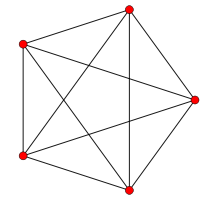5-cell
Pentatope
4-simplex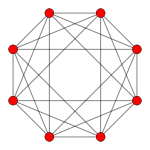16-cell
Orthoplex
4-orthoplex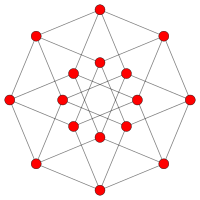8-cell
Tesseract
4-cube
{3,4,3} {5,3,3} {3,3,5}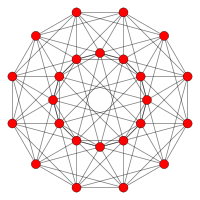Octaplex
24-cell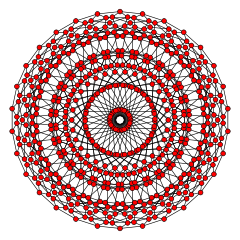Dodecaplex
120-cell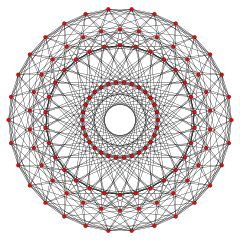Tetraplex
600-cell

In geometry, a 4-polytope (sometimes also called a polychoron, polycell, or polyhedroid) is a four-dimensional polytope. It is a connected and closed figure, composed of lower-dimensional polytopal elements: vertices, edges, faces (polygons), and cells (polyhedra). Each face is shared by exactly two cells.

The two-dimensional analogue of a 4-polytope is a polygon, and the three-dimensional analogue is a polyhedron.

Topologically 4-polytopes are closely related to the uniform honeycombs, such as the cubic honeycomb, which tessellate 3-space; similarly the 3D cube is related to the infinite 2D square tiling. Convex 4-polytopes can be cut and unfolded as nets in 3-space.

## Definition

A 4-polytope is a closed four-dimensional figure. It comprises vertices (corner points), edges, faces and cells. A cell is the three-dimensional analogue of a face, and is therefore a polyhedron. Each face must join exactly two cells, analogous to the way in which each edge of a polyhedron joins just two faces. Like any polytope, the elements of a 4-polytope cannot be subdivided into two or more sets which are also 4-polytopes, i.e. it is not a compound.

The most familiar 4-polytope is the tesseract or hypercube, the 4D analogue of the cube.

## Visualisation

Example presentations of a 24-cell
Sectioning Net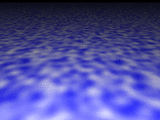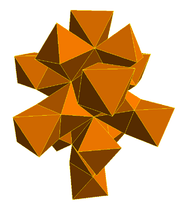Projections
Schlegel 2D orthogonal 3D orthogonal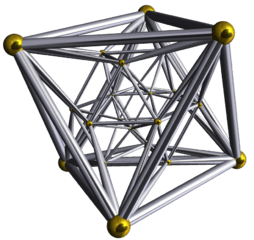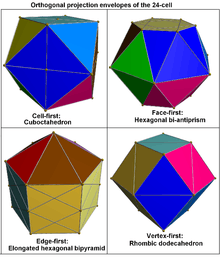4-polytopes cannot be seen in three-dimensional space due to their extra dimension. Several techniques are used to help visualise them.

Orthogonal projection

Orthogonal projections can be used to show various symmetry orientations of a 4-polytope. They can be drawn in 2D as vertex-edge graphs, and can be shown in 3D with solid faces as visible projective envelopes.

Perspective projection

Just as a 3D shape can be projected onto a flat sheet, so a 4-D shape can be projected onto 3-space or even onto a flat sheet. One common projection is a Schlegel diagram which uses stereographic projection of points on the surface of a 3-sphere into three dimensions, connected by straight edges, faces, and cells drawn in 3-space.

Sectioning

Just as a slice through a polyhedron reveals a cut surface, so a slice through a 4-polytope reveals a cut "hypersurface" in three dimensions. A sequence of such sections can be used to build up an understanding of the overall shape. The extra dimension can be equated with time to produce a smooth animation of these cross sections.

Nets

A net of a 4-polytope is composed of polyhedral cells that are connected by their faces and all occupy the same three-dimensional space, just as the polygon faces of a net of a polyhedron are connected by their edges and all occupy the same plane.

## Topological characteristics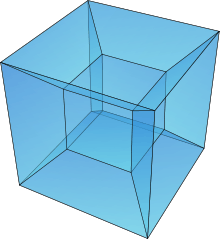The topology of any given 4-polytope is defined by its Betti numbers and torsion coefficients.

The value of the Euler characteristic used to characterise polyhedra does not generalize usefully to higher dimensions, and is zero for all 4-polytopes, whatever their underlying topology. This inadequacy of the Euler characteristic to reliably distinguish between different topologies in higher dimensions led to the discovery of the more sophisticated Betti numbers.

Similarly, the notion of orientability of a polyhedron is insufficient to characterise the surface twistings of toroidal 4-polytopes, and this led to the use of torsion coefficients.

## Classification

### Criteria

Like all polytopes, 4-polytopes may be classified based on properties like "convexity" and "symmetry".

### Classes

The following lists the various categories of 4-polytopes classified according to the criteria above: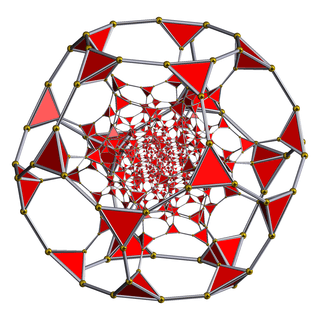The truncated 120-cell is one of 47 convex non-prismatic uniform 4-polytopes

Other convex 4-polytopes: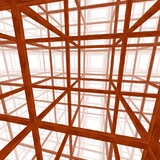The regular cubic honeycomb is the only infinite regular 4-polytope in Euclidean 3-dimensional space.

Infinite uniform 4-polytopes of Euclidean 3-space (uniform tessellations of convex uniform cells)

Infinite uniform 4-polytopes of hyperbolic 3-space (uniform tessellations of convex uniform cells)

• 41 unique dual convex uniform 4-polytopes
• 17 unique dual convex uniform polyhedral prisms
• infinite family of dual convex uniform duoprisms (irregular tetrahedral cells)
• 27 unique convex dual uniform honeycombs, including:

Others: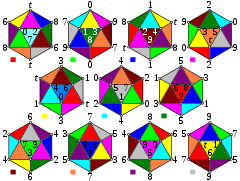The 11-cell is an abstract regular 4-polytope, existing in the real projective plane, it can be seen by presenting its 11 hemi-icosahedral vertices and cells by index and color.

These categories include only the 4-polytopes that exhibit a high degree of symmetry. Many other 4-polytopes are possible, but they have not been studied as extensively as the ones included in these categories.

• Regular 4-polytope
• The 3-sphere (or glome) is another commonly discussed figure that resides in 4-dimensional space. This is not a 4-polytope, since it is not bounded by polyhedral cells.
• The duocylinder is a figure in 4-dimensional space related to the duoprisms. It is also not a 4-polytope because its bounding volumes are not polyhedral.

## References

### Notes

1. Vialar, T. (2009). Complex and Chaotic Nonlinear Dynamics: Advances in Economics and Finance. Springer. p. 674. ISBN 978-3-540-85977-2.
2. Capecchi, V.; Contucci, P.; Buscema, M.; D'Amore, B. (2010). Applications of Mathematics in Models, Artificial Neural Networks and Arts. Springer. p. 598. doi:10.1007/978-90-481-8581-8. ISBN 978-90-481-8580-1.
3. Richeson, D.; Euler's Gem: The Polyhedron Formula and the Birth of Topoplogy, Princeton, 2008.
4. Uniform Polychora, Norman W. Johnson (Wheaton College), 1845 cases in 2005

### Bibliography

• H.S.M. Coxeter:
• H. S. M. Coxeter, M. S. Longuet-Higgins and J. C. P. Miller: Uniform Polyhedra, Philosophical Transactions of the Royal Society of London, Londne, 1954
• H.S.M. Coxeter, Regular Polytopes, 3rd Edition, Dover New York, 1973
• Kaleidoscopes: Selected Writings of H.S.M. Coxeter, edited by F. Arthur Sherk, Peter McMullen, Anthony C. Thompson, Asia Ivic Weiss, Wiley-Interscience Publication, 1995, ISBN 978-0-471-01003-6
• (Paper 22) H.S.M. Coxeter, Regular and Semi Regular Polytopes I, [Math. Zeit. 46 (1940) 380–407, MR 2,10]
• (Paper 23) H.S.M. Coxeter, Regular and Semi-Regular Polytopes II, [Math. Zeit. 188 (1985) 559–591]
• (Paper 24) H.S.M. Coxeter, Regular and Semi-Regular Polytopes III, [Math. Zeit. 200 (1988) 3–45]
• J.H. Conway and M.J.T. Guy: Four-Dimensional Archimedean Polytopes, Proceedings of the Colloquium on Convexity at Copenhagen, page 38 und 39, 1965
• N.W. Johnson: The Theory of Uniform Polytopes and Honeycombs, Ph.D. Dissertation, University of Toronto, 1966
• Four-dimensional Archimedean Polytopes (German), Marco Möller, 2004 PhD dissertationWikimedia Commons has media related to Polychora.

Fundamental convex regular and uniform polytopes in dimensions 2–10
Family An Bn I2(p) / Dn E6 / E7 / E8 / E9 / E10 / F4 / G2 Hn
Regular polygon Triangle Square p-gon Hexagon Pentagon
Uniform polyhedron Tetrahedron OctahedronCube Demicube DodecahedronIcosahedron
Uniform 4-polytope 5-cell 16-cellTesseract Demitesseract 24-cell 120-cell600-cell
Uniform 5-polytope 5-simplex 5-orthoplex5-cube 5-demicube
Uniform 6-polytope 6-simplex 6-orthoplex6-cube 6-demicube 122221
Uniform 7-polytope 7-simplex 7-orthoplex7-cube 7-demicube 132231321
Uniform 8-polytope 8-simplex 8-orthoplex8-cube 8-demicube 142241421
Uniform 9-polytope 9-simplex 9-orthoplex9-cube 9-demicube
Uniform 10-polytope 10-simplex 10-orthoplex10-cube 10-demicube
Uniform n-polytope n-simplex n-orthoplexn-cube n-demicube 1k22k1k21 n-pentagonal polytope
Topics: Polytope familiesRegular polytopeList of regular polytopes and compounds